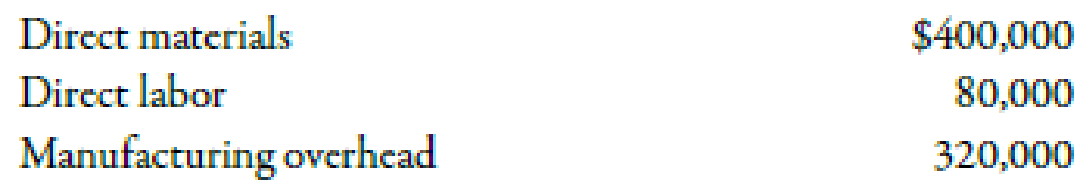Chapter 2, Problem 42E### Managerial Accounting: The Corners...

7th Edition
Maryanne M. Mowen + 2 others
ISBN: 9781337115773

#### Solutions

Chapter
Section### Managerial Accounting: The Corners...

7th Edition
Maryanne M. Mowen + 2 others
ISBN: 9781337115773
Textbook Problem
31 views

# Use the following information for Exercises 2-41 and 2-42:Grin Company manufactures digital cameras. In January, Grin produced 4,000 cameras with the following costs:There were no beginning or ending inventories of WIP.Exercise 2-42 Prime Cost and Conversion CostRefer to the information for Grin Company above.Required: 1. What was the total prime cost in January? 2. What was the prime cost per unit in January? 3. What was the total conversion cost in January? 4. What was the conversion cost per unit in January?

1.

To determine

Calculate the amount of total prime cost in January.

Explanation

Prime Cost:

Prime cost can be defined as the summation of direct labor cost and direct material cost. The amount of indirect cost is not included while evaluating the prime cost.

Use the following formula to compute the total prime cost:

Total prime cost=Direct material+Direct labor

Substitute $400,000 for direct material and$80,000 for direct labor in the above formula

2.

To determine

Calculate the amount of prime cost per unit in January.

3.

To determine

Calculate the amount of total conversion cost in January.

4.

To determine

Calculate the amount of conversion cost per unit in January.

### Still sussing out bartleby?

Check out a sample textbook solution.

See a sample solution

#### The Solution to Your Study Problems

Bartleby provides explanations to thousands of textbook problems written by our experts, many with advanced degrees!

Get Started